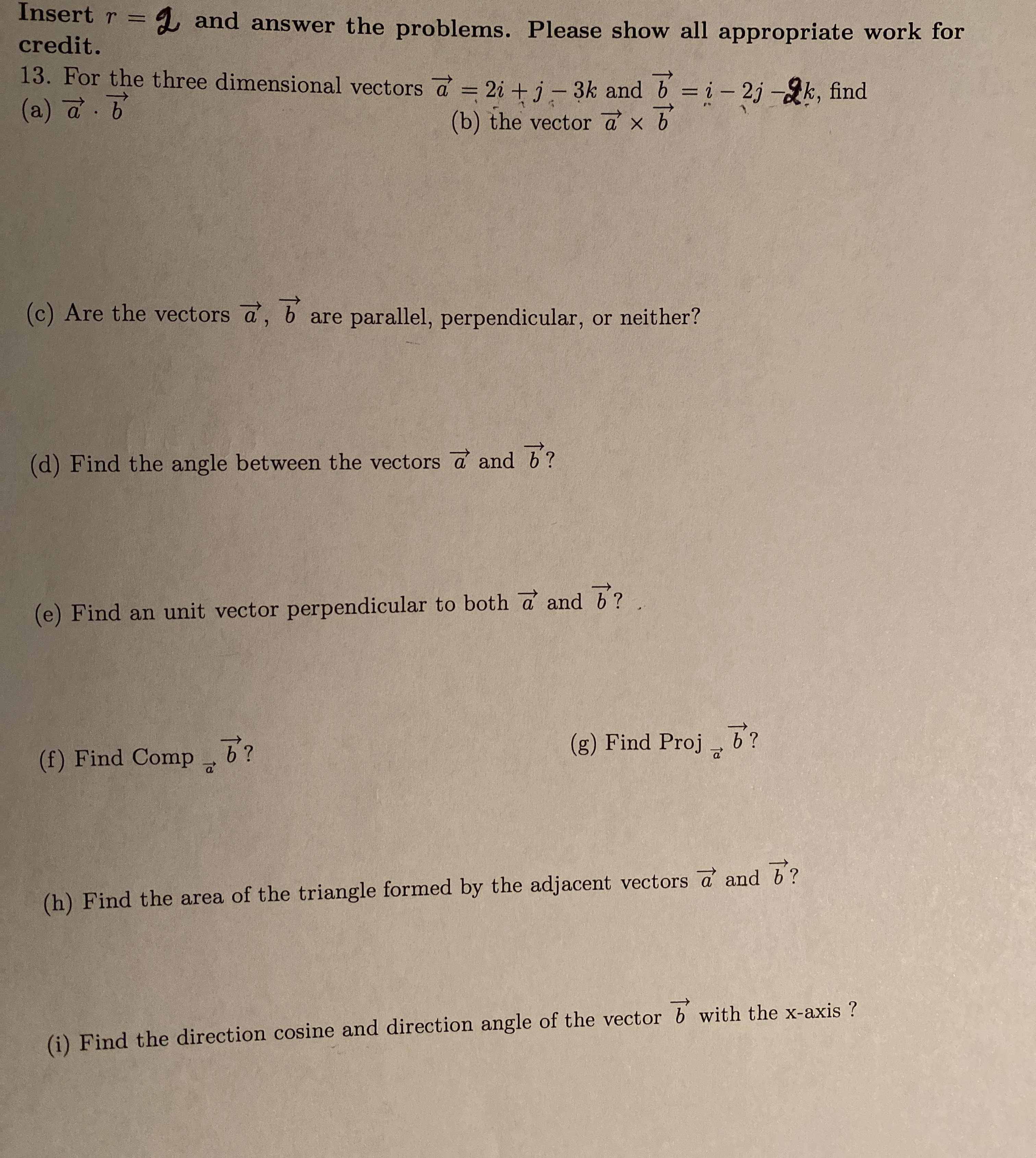# Insert rcredit.and answer the problems. Please show all appropriate work for13. For the three dimensional vectors a 2i + j-3k and b = i- 2j -k, find(a) a. b(b) the vector a x b(c) Are the vectors a, b are parallel, perpendicular, or neither?(d) Find the angle between the vectors a and b?(e) Find an unit vector perpendicular to both a and b?(g) Find Proj?(f) Find Comp(h) Find the area of the triangle formed by the adjacent vectors a and b?with the x-axis ?(i) Find the direction cosine and direction angle of the vector b

Question
4 viewshelp_outlineImage TranscriptioncloseInsert r credit. and answer the problems. Please show all appropriate work for 13. For the three dimensional vectors a 2i + j-3k and b = i- 2j -k, find (a) a. b (b) the vector a x b (c) Are the vectors a, b are parallel, perpendicular, or neither? (d) Find the angle between the vectors a and b? (e) Find an unit vector perpendicular to both a and b? (g) Find Proj ? (f) Find Comp (h) Find the area of the triangle formed by the adjacent vectors a and b? with the x-axis ? (i) Find the direction cosine and direction angle of the vector b fullscreen
check_circle

Step 1

Since we only answer up to 3 sub-parts, we’ll answer the first 3. Please resubmit the question and specify the other subparts (up to 3) you’d like answered.

To determine:

Part a) Scalar product of a and b.

Part b) Vector product of a and b.

Part c) If the vectors a and b are parallel, perpendicular or neither.

Step 2

Given:

Step 3

Part a)

...

### Want to see the full answer?

See Solution

#### Want to see this answer and more?

Solutions are written by subject experts who are available 24/7. Questions are typically answered within 1 hour.*

See Solution
*Response times may vary by subject and question.
Tagged in

### Other